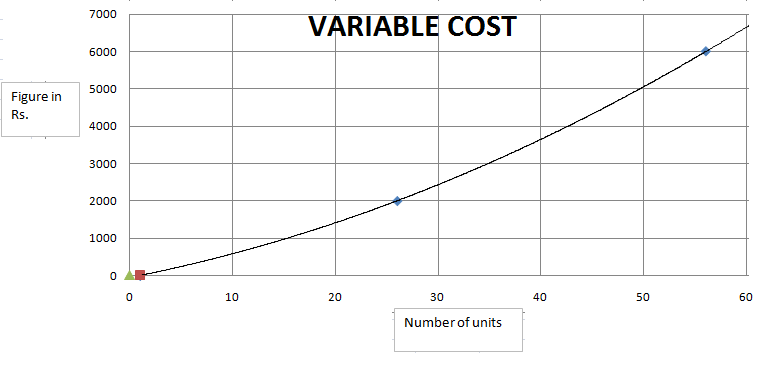### CAT 2000 Question Paper Question 36

Instructions

Answer these questions based on the following Information:

ABC Ltd. produces widgets for which the demand is unlimited and they can sell all of their production. The graph below describes the monthly variable costs incurred by the company as a function of the quantity produced. In addition, operating the plant for one shift results in a fixed monthly cost of Rs. 800. Fixed monthly costs for second shift operation are estimated at Rs. 1200. Each shift operation provides capacity for producing 30 widgets per month.
Note: Average unit cost, AC = Total monthly costs/monthly production, and Marginal cost MC is the rate of change in total cost for unit change in quantity produced.Question 36

# Assume that the unit price is Rs. 150 and profit is defined as the excess of sales revenue over total costs. What is the monthly production level of ABC Ltd. at which the profit is highest?

Solution

As adding a shift dramatically increases the costs, we need to consider the two regions, 0<x<=30 and 30<x<=60, separately.

Region 1: As variable cost is less than equal to 100, and sales price is 150, profit would be max when production is maximum possible i.e. at 30 units.

Hence, at 30 units, total cost = 800 + 2400 = 3200. Sales = 30 * 150 = 4500. Profit = 4500-3200 = 1300

Region 2: As variable cost is less than equal to 100, and sales price is 150, profit would be max when production is maximum possible i.e. at 60 units

Hence, at 60 units, total cost = 800 + 1200 + 6500 = 8500. Sales = 150*60 = 9000. Hence, Profit = 9000 - 8500 = 500

Hence, profit is maximum at 30 units.